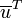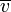# Hessian matrix defines bilinear form that outputs second-order directional derivatives

Suppose$f$ is a function of$n$ variables$x_1,x_2,\dots,x_n$, which we think of as a vector variable$\overline{x}$. Suppose$\overline{u},\overline{v}$ are unit vectors in$n$-space. Then, we have the following:$D_{\overline{v}}(D_{\overline{u}}(f)) = \overline{u}^TH(f)\overline{v}$
where$\overline{u},\overline{v}$ are treated as column vectors, so$\overline{u}^T$ is$\overline{u}$ as a row vector, and$\overline{v}$ is$\overline{v}$ as a column vector. The multiplication on the right side is matrix multiplication. Note that this tells us that the bilinear form corresponding to the Hessian matrix outputs second-order directional derivatives.$D_{\overline{v}}(D_{\overline{u}}(f)) = D_{\overline{u}}(D_{\overline{v}}(f))$